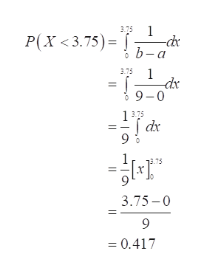# The waiting times between a subway departure schedule and the arrival of a passenger are uniformly distributed between 0 and 9 minutes. Find the probability that a randomly selected passenger has a waiting time less than 3.75 minutes. (round to three decimal places)

Question
188 views

The waiting times between a subway departure schedule and the arrival of a passenger are uniformly distributed between 0 and 9 minutes. Find the probability that a randomly selected passenger has a waiting time less than 3.75 minutes. (round to three decimal places)

check_circle

Step 1

A uniform distribution has an equal probability for all the outcomes of the random variable, that is, it has a constant probability. A continuous uniform distribution is defined over the range (a, b) where a is the smallest value and b is the largest value of the distribution. The values (a, b), are the parameters of the uniform distribution.

Consider, X be the random variable that represents the passengers waiting time is uniformly distributed with the parameters a= 0 and b = 9.

Step 2

The probability that a randomly selected passenger has a wait...help_outlineImage Transcriptionclose1 P(X 3.75)= 375 b-a 375 1 dx 9-0 1375 dx 3.75-0 = 0.417 fullscreen

### Want to see the full answer?

See Solution

#### Want to see this answer and more?

Solutions are written by subject experts who are available 24/7. Questions are typically answered within 1 hour.*

See Solution
*Response times may vary by subject and question.
Tagged in

### Other